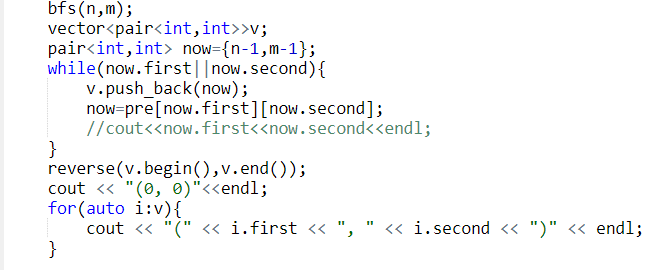#### 指针原来是套娃的等　　级 排　　名 10 经　　验 23641 参赛次数 49 文章发表 123 年　　龄 0 在职情况 学生 学　　校 专　　业 物联网工程

自我简介：

QQ：2830671713

#### TA的其他文章

 2892: 最好的草浏览：15 1192: 十->二进制转换（位运算做法）浏览：29

#### 你可能喜欢#include <bits/stdc++.h>
#include <queue>
using namespace std;

const int S=5;

struct node{
int x,y;
string s; //存放位置
};

int dx={1,0,0,-1};
int dy={0,-1,1,0};

bool vis[S][S];
int p[S][S];
queue<node>q;

void bfs(int n,int m){
q.push(node{0,0}); //开始在0,0处

while(!q.empty()){

node now=q.front();//获取队首位置
vis[now.x][now.y]=1;
q.pop();

if(now.x==n-1&&now.y==m-1){
for(int i=0;i<now.s.size();i+=2){//打印
cout<<"("<<now.s[i]<<", "<<now.s[i+1]<<")"<<endl;
}
cout<<"(4, 4)";//因为不存放自身的位置，所以需要单独打印
break;
}
for(int i=0;i<4;i++){
int x=now.x+dx[i];
int y=now.y+dy[i];
if(x>=0&&y>=0&&x<n&&y<m){//不超过数组大小
if(p[x][y]==0&&vis[x][y]==0){//可以走且没有走过
vis[x][y]=1;
node nom=now;//现在num.s已经存放了now.s记录的位置（核心代码）
nom.x=x;
nom.y=y;
nom.s+=now.x+'0';//将上一步的位置坐标新增到s里面（核心代码）
nom.s+=now.y+'0';
q.push(nom);
}
}
}
}
}

int main()
{
int n=5,m=5;
for(int i=0;i<n;i++){
for(int j=0;j<m;j++)cin>>p[i][j];
}

bfs(n,m);
return 0;
}

0.0分

5 人评分

#### 评论区

强！
2023-03-17 22:11:33
• «
• 1
• »